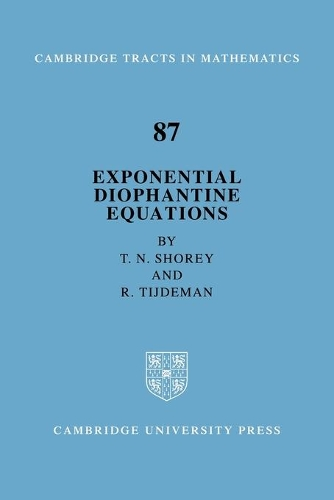•Cambridge Tracts in Mathematics: Exponential Diophantine Equations Series Number 87 (Paperback)

(author), (author)
£44.99
Paperback 252 Pages / Published: 04/12/2008
• Not available

This product is currently unavailable.

This is a integrated presentation of the theory of exponential diophantine equations. The authors present, in a clear and unified fashion, applications to exponential diophantine equations and linear recurrence sequences of the Gelfond-Baker theory of linear forms in logarithms of algebraic numbers. Topics covered include the Thue equations, the generalised hyperelliptic equation, and the Fermat and Catalan equations. The necessary preliminaries are given in the first three chapters. Each chapter ends with a section giving details of related results.

Publisher: Cambridge University Press
ISBN: 9780521091701
Number of pages: 252
Weight: 380 g
Dimensions: 229 x 152 x 15 mm